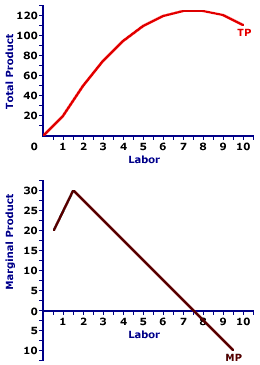Monday  September 25, 2023
 AmosWEB means Economics with a Touch of Whimsy!RATE OF RETURN: The ratio of the additional annual income or profit generated by an investment to the cost of the investment. Here's a simple example, although the calculations are usually a great deal more involved for actual investments. If the cost of constructing a new factory is \$10 million and it gives you an extra \$1 million in profit each year, then its rate of return is 10 percent.TOTAL PRODUCT AND MARGINAL PRODUCT:

A mathematical connection between marginal product and total product stating that marginal product IS the slope of the total product curve. If the total product curve has a positive slope (that is, is upward sloping), then marginal product is positive. If the total product curve has a negative slope (downward sloping), then marginal product is negative. If the total product curve has a zero slope (horizontal), then marginal product is zero.
The relation between total product and marginal product is one of several that reflect the general relation between a total and the corresponding marginal. There general relation is this: A marginal is the slope of the total curve. Marginal is another term for slope.

The general relation between total and marginal surfaces throughout the study of economics, especially utility (total utility and marginal utility), cost (total cost and marginal cost), revenue (total revenue and marginal revenue), and consumption (consumption expenditures and marginal propensity to consume).

### Making Tacos

Total and Marginal ProductThis two-paneled graph for the hourly production of Super Deluxe TexMex Gargantuan Tacos (with sour cream and jalapeno peppers) that visually illustrates the connection between total product and marginal product.
• For the first few quantities of the variable input (the number of workers), total product in the top panel is positive AND the slope of the total product curve increases, it becomes steeper. This corresponds with a positive and increasing marginal product in the bottom panel.

• For the next several quantities of the variable input, the slope of the total product curve flattens out, although positive, the slope decreases. This corresponds to a decreasing marginal product in the bottom panel.

• For the last few quantities of the variable input, the slope of the total product curve in the top panel is negative. This corresponds with a negative marginal product in the bottom panel.

### The Law of Diminishing Marginal Returns

The prime conclusion to drawn from this is the key role played by the law of diminishing marginal returns in the slope of both the marginal product curve and the total product curve. The "hump shape" of the marginal product curve is a direct reflection of first increasing marginal returns, as marginal product rises to a peak, then decreasing marginal returns and the onset of the law of diminishing marginal returns as marginal product falls.

However, because the marginal product curve is essentially a plot of the slope of the total product curve, the shape of the total product curve also reflects the law of diminishing marginal returns. The increasingly steep slope of the total product curve for small quantities of the variable input is due to increasing marginal returns. Then with the onset of the law of diminishing marginal returns causes the total product curve to flatten out and subsequently turn down.

 <= TOTAL PRODUCT AND AVERAGE PRODUCT TOTAL PRODUCT CURVE =>Recommended Citation:

TOTAL PRODUCT AND MARGINAL PRODUCT, AmosWEB Encyclonomic WEB*pedia, http://www.AmosWEB.com, AmosWEB LLC, 2000-2023. [Accessed: September 25, 2023].

Check Out These Related Terms...

Or For A Little Background...

And For Further Study...
Search Again?PURPLE SMARPHIN[What's This?] Today, you are likely to spend a great deal of time lost in your local discount super center wanting to buy either hand lotion, a big bottle of hand lotion or a lighted magnifying glass. Be on the lookout for malfunctioning pocket calculators.Your Complete ScopeDuring the American Revolution, the price of corn rose 10,000 percent, the price of wheat 14,000 percent, the price of flour 15,000 percent, and the price of beef 33,000 percent."If I'm selecting a group, the first thing I look for is a record of achievement . . . If (candidates achieve) in small things, there's a very good chance they'll perform well in big things. "-- Edmund Hillary, explorerBAEBureau of Agricultural EconomicsA PEDestrian's Guide Xtra CreditTell us what you think about AmosWEB. Like what you see? Have suggestions for improvements? Let us know. Click the User Feedback link.| | | | | | | | | | |
| | | |

Thanks for visiting AmosWEB